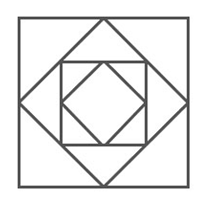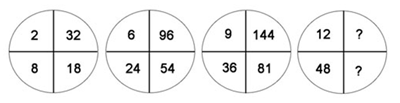# Friday Puzzle

Draw the figure without raising your pencil.# What Next?

Fill in the next line:

9 7 5 9 4 9
6 3 4 5 3 6
1 8 2 0 1 8

_______

# Missing Letters

Find the missing letters which complete the group.

B, C, D, E, ?, P, T, ?

# Complete The Series

B C D E __ I K __ X

The puzzle might look like a simple one. Give it a try.

# Missing Numbers

What are the missing numbers?# Number Puzzle

Make 100 using six 9. You can use addition, division and fraction.

# July Equals To…

If March = 43 and May = 39, what does July equals to?

# Quick and Easy Puzzle

Find the values of  a and b:

12 x a + b = 98

123 x a + b+1 = 987

1234 x a + b+2 = 9876

12345 x a + b+3 = 98765

123456 x a + b+4 = 987654

1234567 x a + b+5 = 9876543

12345678 x a + b+6 = 98765432

123456789 x a + b+7 = 987654321

# What Is The Next Number?

Fill in the sequence:

1   11   21   1211   111221  __________

Looks simple. Try solving it.

Do not post answers. Just write if you could answer it or not and how long did it take to solve.